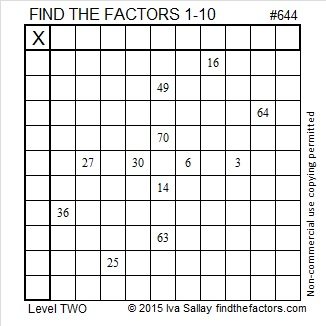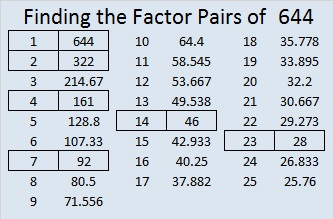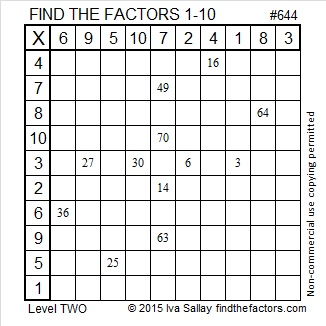# 644 Divisibility Rules and Level 2

Divisibility rules 1-11 applied to the number 644:

1. All counting numbers are divisible by 1 so 644 IS divisible by 1.
2. 644 is even so it IS divisible by 2.
3. 6 + 4 + 4 = 14 which is not a multiple of 3 so 644 is NOT divisible by 3.
4. The last two digits, 44, are divisible by 4, so 644 IS divisible by 4.
5. The last digit is not 0 or 5, so 644 is NOT divisible by 5.
6. 644 is divisible by 2, but not by 3 so 644 is NOT divisible by 6.
7. Breaking off the last digit, doubling it and subtracting it from the remaining digits we get: 64 – 2(4) = 56, a multiple of 7, so 644 IS divisible by 7.
8. 6 is an even digit, and 44 is divisible by 4 but not by 8 so 644 is NOT divisible by 8.
9. 6 + 4 + 4 = 14 which is not a multiple of 9 so 644 is NOT divisible by 9.
10. The last digit is not 0, so 644 is NOT divisible by 10.
11. 6 – 4 + 4 = 6 which is not a multiple of 11 so 644 is NOT a multiple of 11.

Just for the fun of it let’s try a divisibility rule for 23. Break off the last digit, multiply it by 7 and add it to the remaining digits: 64 + 7(4) = 64 + 28 = 92.

Now apply the same rule to 92: 9 + 7(2) = 9 + 14 = 23, obviously a multiple of 23, so 92 and 644 are both divisible by 23.Print the puzzles or type the solution on this excel file: 10 Factors 2015-10-12

—————————————————————————————————

• 644 is a composite number.
• Prime factorization: 644 = 2 x 2 x 7 x 23, which can be written 644 = (2^2) x 7 x 23
• The exponents in the prime factorization are 2, 1, and 1. Adding one to each and multiplying we get (2 + 1)(1 + 1)(1 + 1) = 3 x 2 x 2 = 12. Therefore 644 has exactly 12 factors.
• Factors of 644: 1, 2, 4, 7, 14, 23, 28, 46, 92, 161, 322, 644
• Factor pairs: 644 = 1 x 644, 2 x 322, 4 x 161, 7 x 92, 14 x 46, or 23 x 28
• Taking the factor pair with the largest square number factor, we get √644 = (√4)(√161) = 2√161 ≈ 25.377155—————————————————————————————————This site uses Akismet to reduce spam. Learn how your comment data is processed.